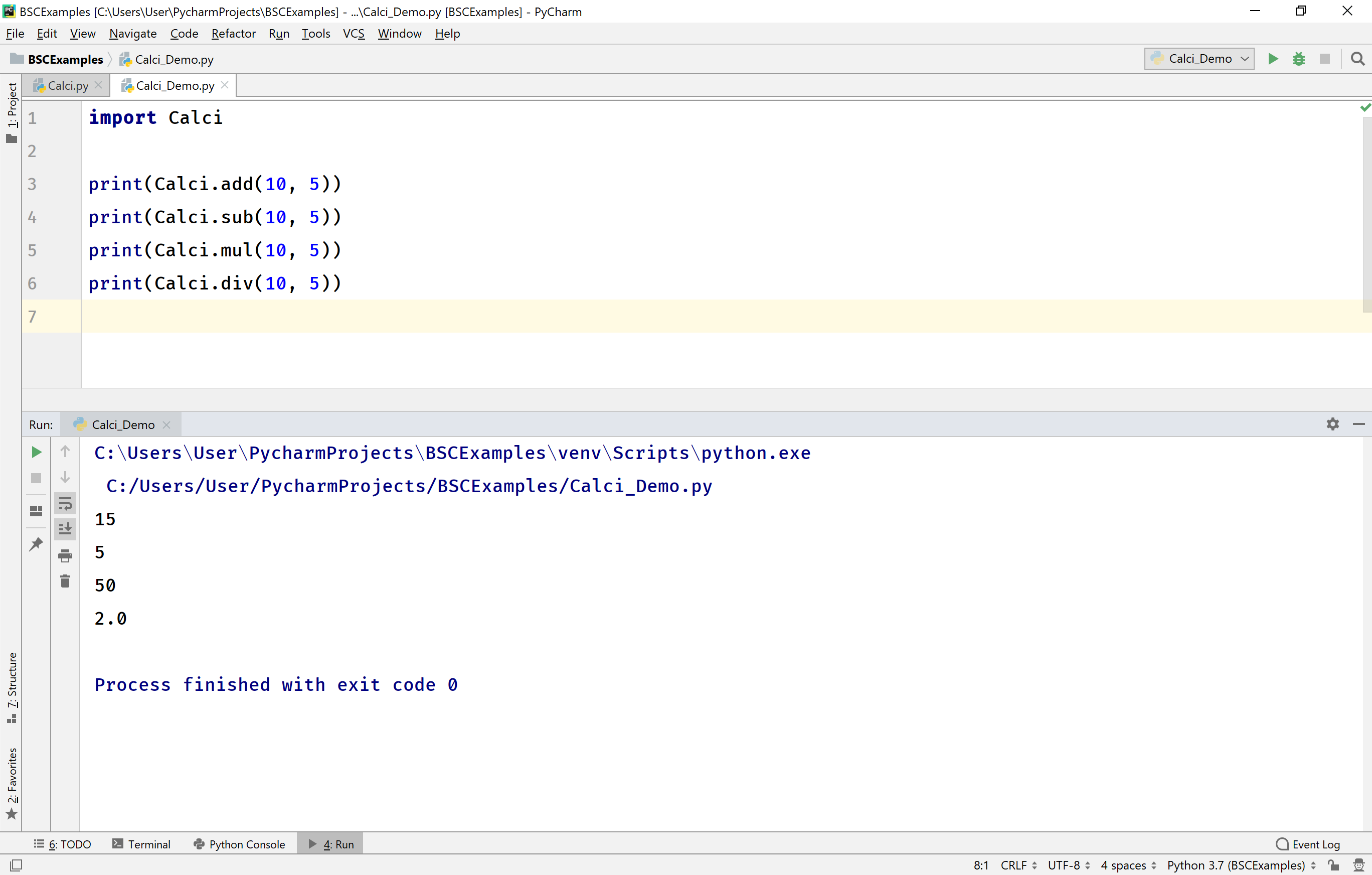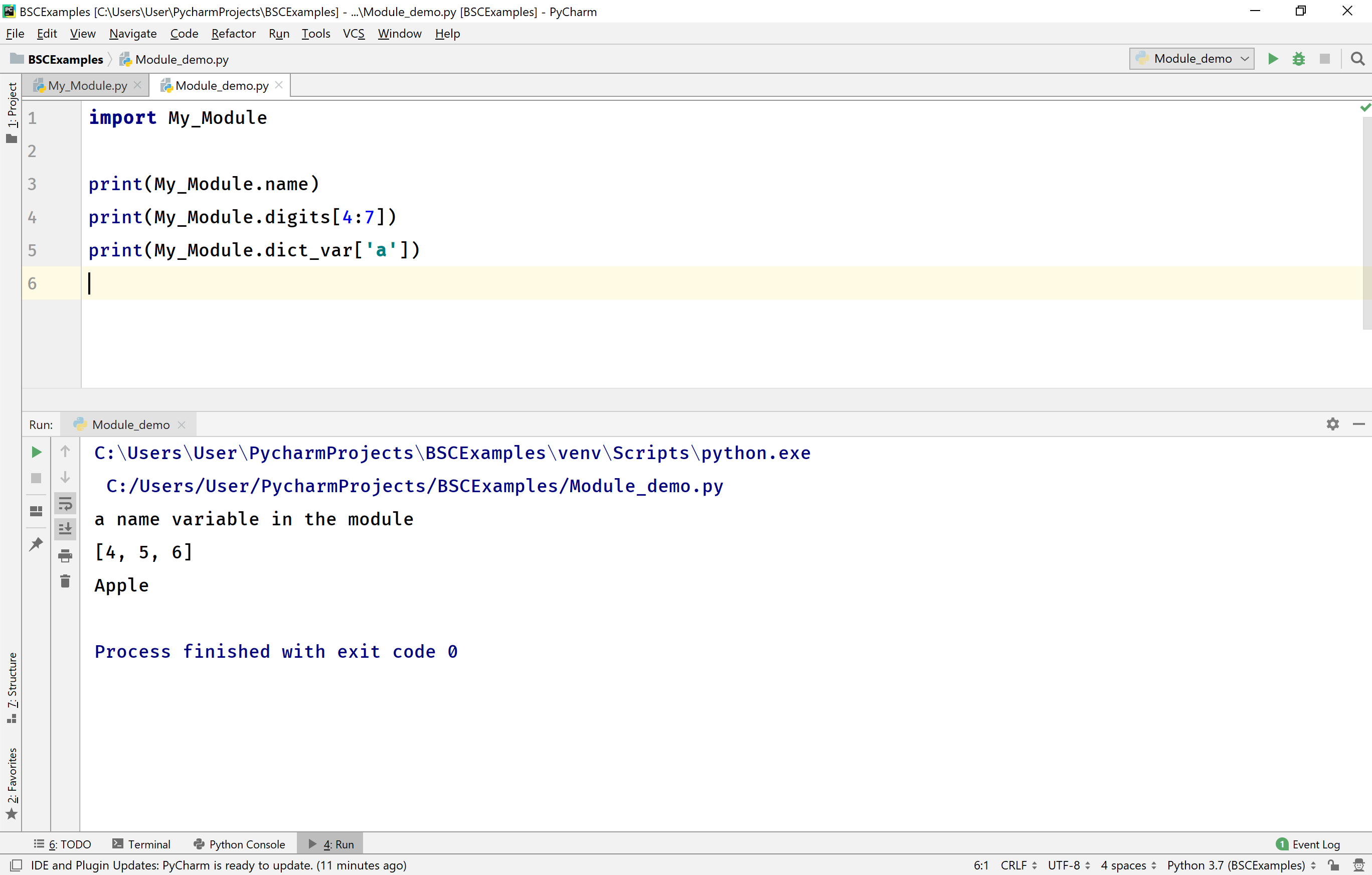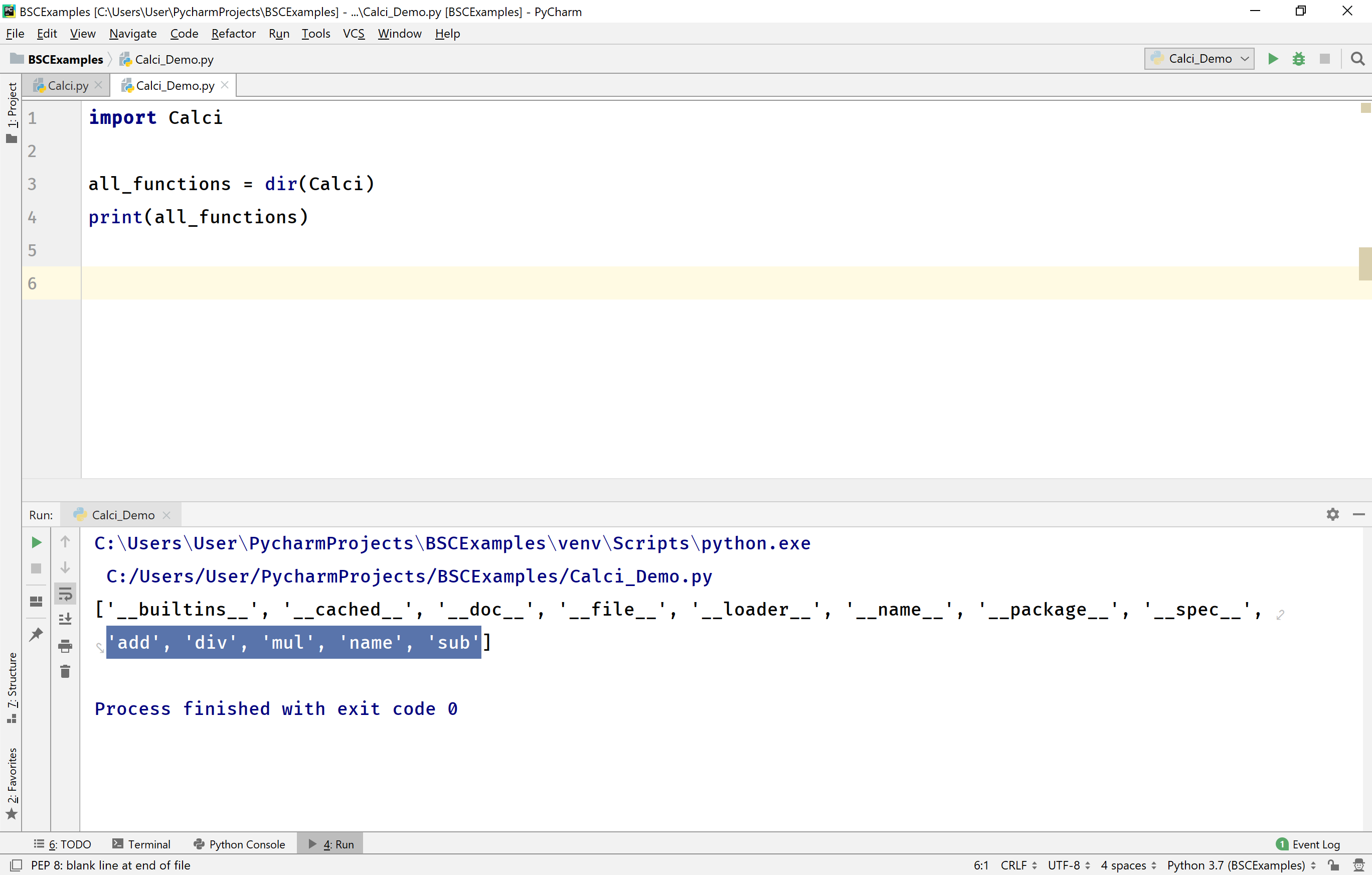The perfect place for easy learning...

×

# Python Modules

In Python, a module is a python file containing functions, class, and variables. A module is included in any python application using an import statement. A python module may also contain runnable python code.
Using python modules, we organize related code into a group which can be included in any python application. The module in python makes the python program easy to understand and use.

# How to create a module?

In Python programming, to create a module simply define the functions, classes, and variables that you want to place in the module and save the file with .py extension. For example, let's create a module which performs all arithmetic operations.

Example
``````def add(a, b):
return a + b

def sub(a, b):
return a - b

def mul(a, b):
return a * b

def div(a, b):
return a / b
``````

Save the above code as Calci.py so that the module can be used as Calci.

# How to use a module?

A module is used in any python application by importing it using the import keyword. Now, let's import the Calci module and use the functions of it.

Example
``````import Calci

print(Calci.sub(10, 5))
print(Calci.mul(10, 5))
print(Calci.div(10, 5))
``````

When we run the above example code, it produces the following output.# Variables in module

The python module may also contain variables. It can contain variable of any type including list, tuples, dictionaries, objects, etc. Let's look at the following module.

Example
``````name = 'a name variable in the module'
digits = [0, 1, 2, 3, 4, 5, 6, 7, 8, 9]
dict_var = {'a':'Apple', 'b':'Ball', 'c':'Cat'}
``````

Save the above file as My_Module.py.
Now, let's import this module and use the variables of it.

Example
``````import My_Module

print(My_Module.name)
print(My_Module.digits[4:7])
print(My_Module.dict_var['a'])
``````

When we run the above example code, it produces the following output.# import and as keywords

In Python, we can import a module with an alias name. To import a module with an alias name we use as a keyword along with import keyword.

Example
``````import Calci as c

print(c.sub(10, 5))
print(c.mul(10, 5))
print(c.div(10, 5))
``````

When we run the above example code, it produces the following output.# importing specific function from a module

In Python, we can also import only specific functions from a module. To import only specific functions from a module, we use from keyword along with import keyword. Let's look at the following example.

Example
``````from Calci import add, mul

#print(sub(10, 5))
print(mul(10, 5))
#print(div(10, 5))
``````

When we run the above example code, it produces the following output.When we import specific functions using from a keyword, it not required to use the module name to call the function instead function is called directly. For example, add(10, 5), but not Calci.add(10,5).

We may also import all the functions of a module using from keyword along with *. For example, from Calci import *.

# dir( ) with modules

In Python, the built-in function dir( ) used to list all the functions of a module. This built-in function can be used with any module. Let's look at the following example.

Example
``````import Calci

all_functions = dir(Calci)
print(all_functions)
``````

When we run the above example code, it produces the following output.The dir( ) function returns a list of all functions of the specified module. The list is included with all the user-defined functions and also built-in function.